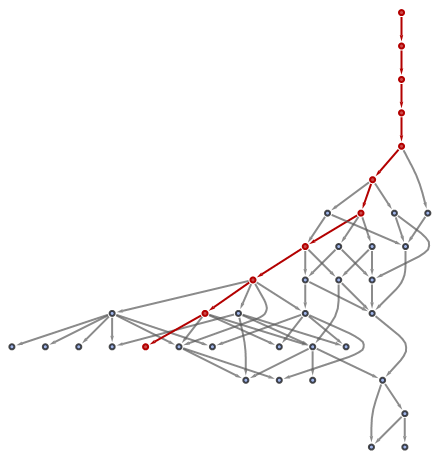#Function Repository Resource:

# FindCombinatorProof

Attempt to find a proof of a theorem in combinatory logic using a given combinatory calculus

Contributed by: Jonathan Gorard
 ResourceFunction["FindCombinatorProof"][thm,<|"Combinators"→comb,"Rules"→rules|>] tries to find an equational proof of the combinatory logic theorem thm using the combinators comb and reduction rules rules. ResourceFunction["FindCombinatorProof"][thm,calc] tries to find an equational proof of the combinatory logic theorem thm using the named combinatory calculus calc.

## Details and Options

If ResourceFunction["FindCombinatorProof"][thm,calc] succeeds in deriving the theorem thm from the combinatory calculus calc, then it returns a ProofObject expression. If it succeeds in showing that the theorem cannot be derived from the combinatory calculus, it returns a Failure object. Otherwise, it may not terminate unless time constrained.
ResourceFunction["FindCombinatorProof"] accepts both standard (named) combinatory calculi and arbitrary calculi specified via combinator reduction rules.
ResourceFunction["FindCombinatorProof"] accepts both individual theorems and lists of theorems.
ResourceFunction["FindCombinatorProof"] has the following options:
 how much time to allow
If ResourceFunction["FindCombinatorProof"] exceeds the specified time constraint, it returns a Failure object.
ResourceFunction["FindCombinatorProof"][thm,calc] uses the Knuth-Bendix completion algorithm to prove that the theorem thm follows from the reduction rules of the combinatory calculus calc.

## Examples

### Basic Examples (3)

Prove an elementary theorem about the single-variable fixed-point (y) combinator, with the reduction rule explicitly specified:

 In:=Out=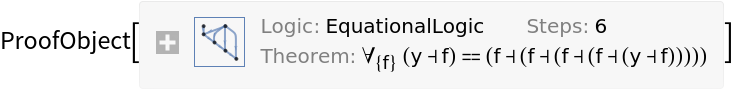Show the abstract proof network, with tooltips showing the intermediate expressions:

 In:=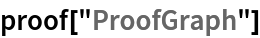Out=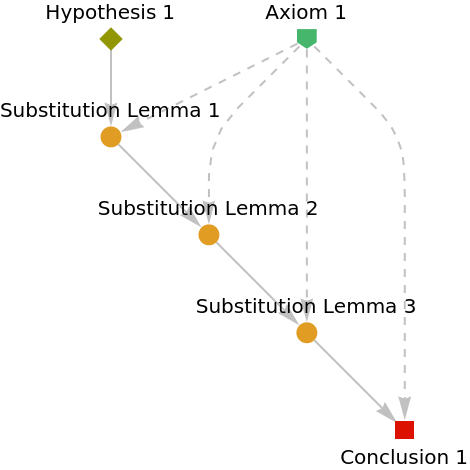Show the complete list of proof steps as a Dataset object:

 In:=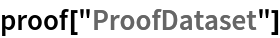Out=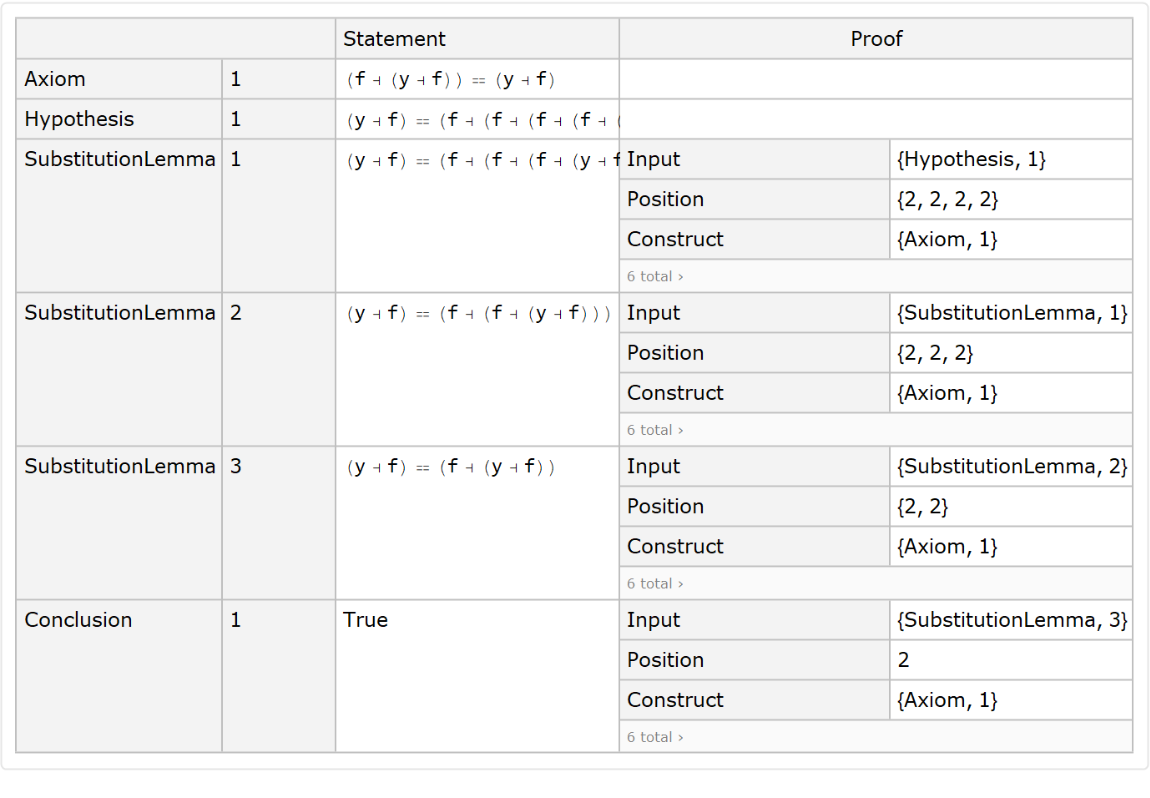Typeset a natural language argument:

 In:=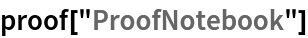Out=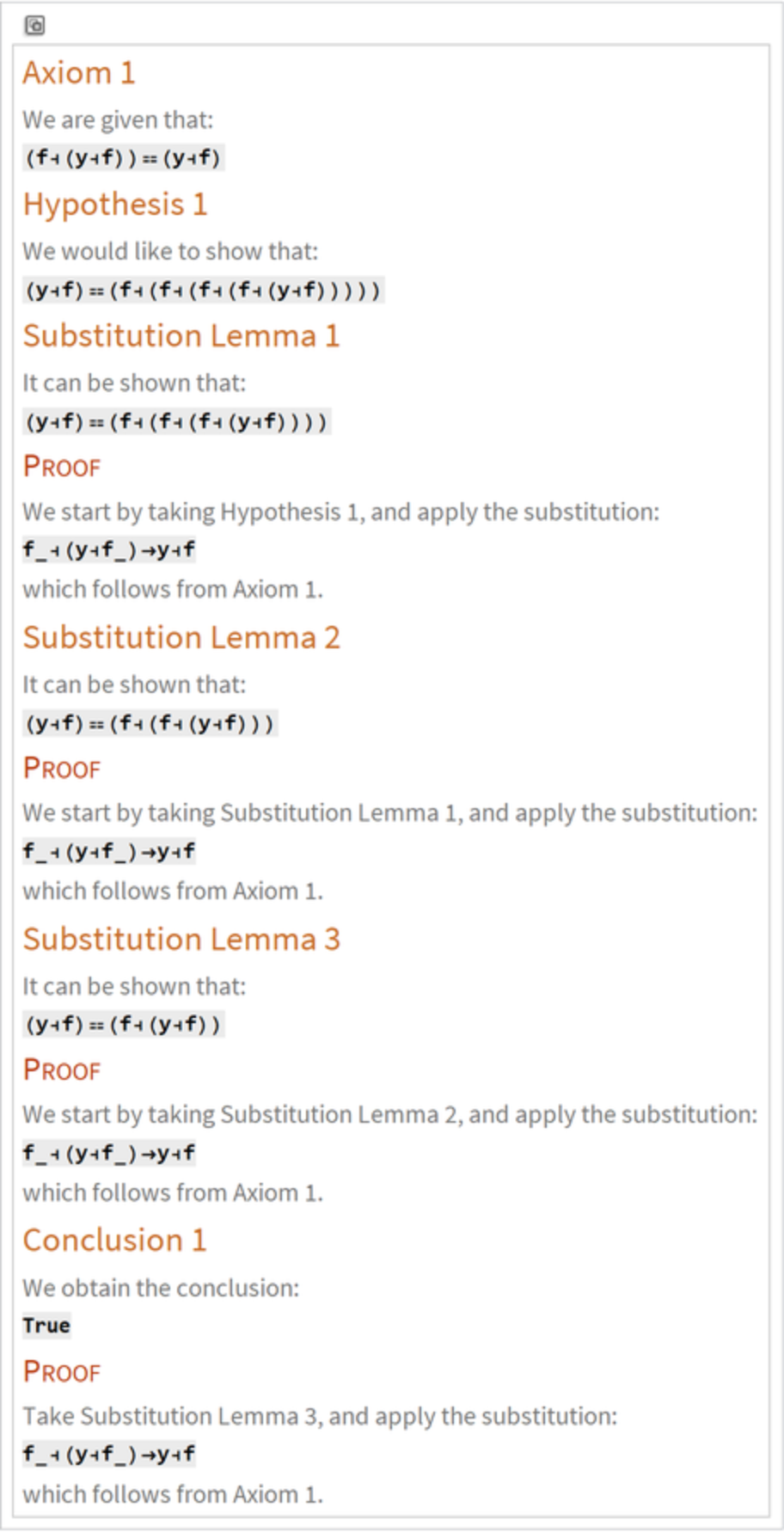Prove a more sophisticated theorem involving the S-K combinatory calculus:

 In:=Out=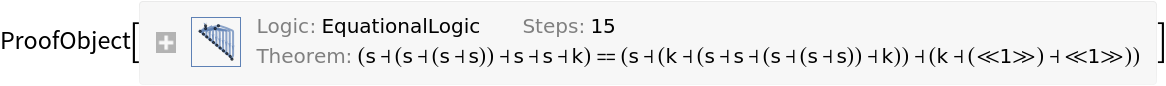Show the abstract proof network:

 In:=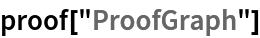Out=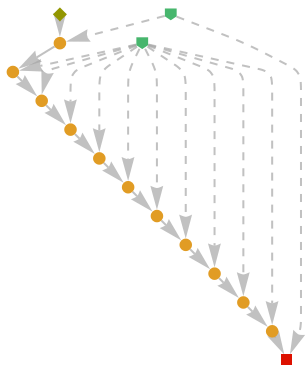Prove a theorem using one of FindCombinatorProof's built-in combinatory calculi (Schönfinkel's B-C-I calculus):

 In:=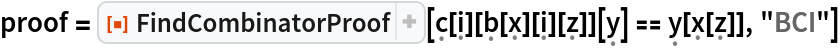Out=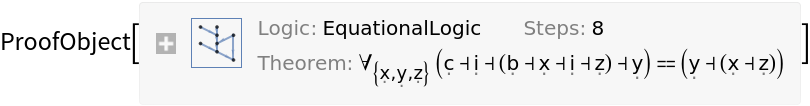Show the abstract proof network:

 In:=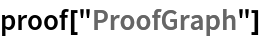Out=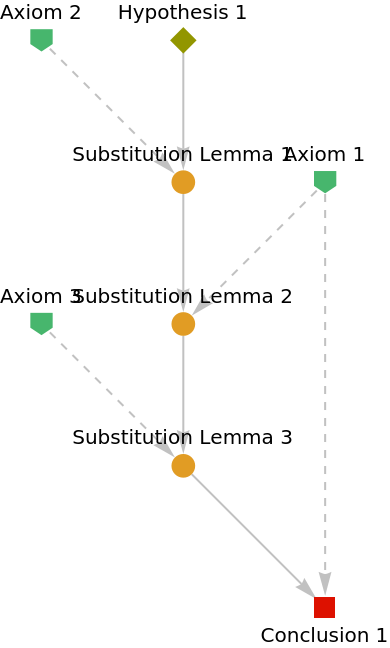### Scope (7)

Show that a combinator equivalence proposition cannot be derived from a given combinatory calculus:

 In:=Out=#### Named Combinatory Calculi (6)

FindCombinatorProof supports a variety of named combinatory calculi, including the single-variable fixed-point (y) combinator:

 In:=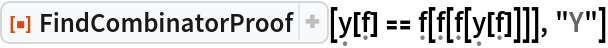Out=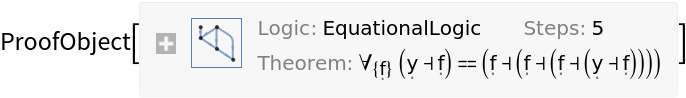The two-variable fixed-point (y) combinator:

 In:=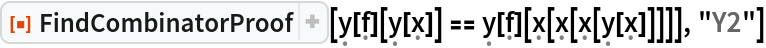Out=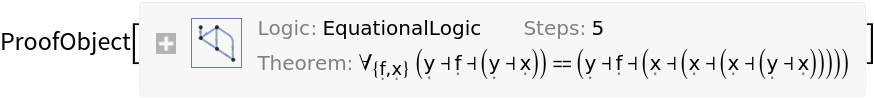The S-K calculus:

 In:=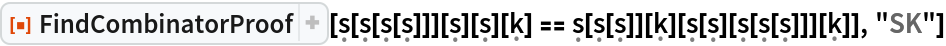Out=The S-K-I calculus:

 In:=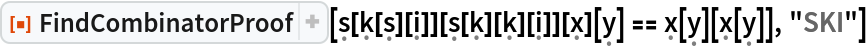Out=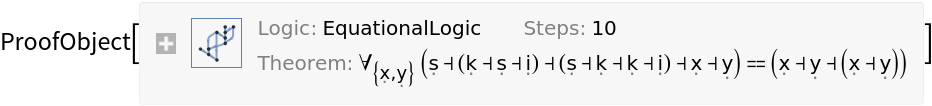Schönfinkel's B and C combinators:

 In:=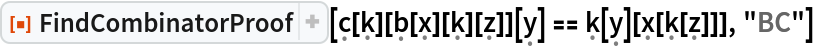Out=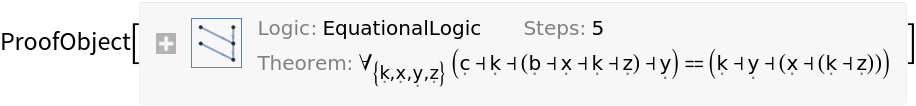The B-C-I calculus:

 In:=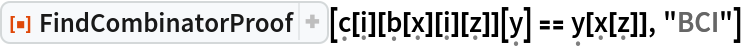Out=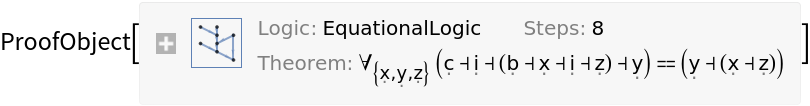#### Initial Conditions (1)

FindCombinatorProof accepts both individual theorems and lists of theorems:

 In:=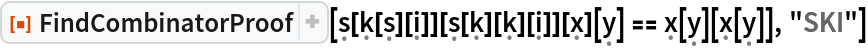Out=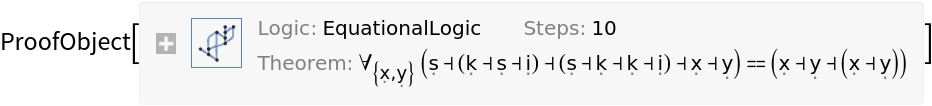In:=Out=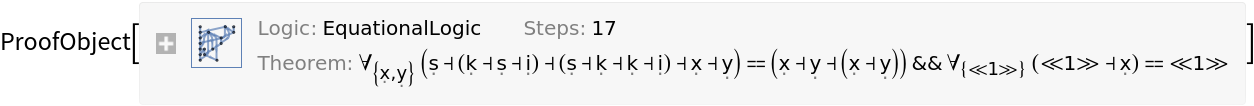### Options (1)

#### TimeConstraint (1)

Use to limit the computation time to t seconds:

 In:=Out=In:=Out=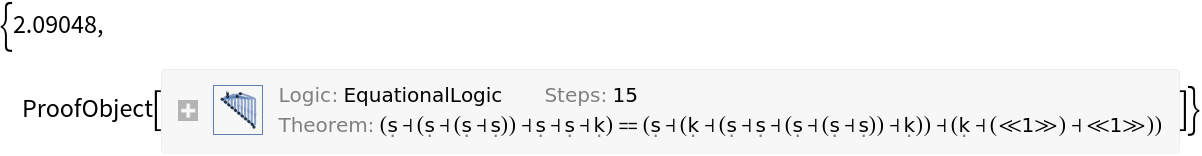### Properties and Relations (1)

FindCombinatorProof will return a proof object for a particular theorem if and only if the associated path exists in the corresponding multiway system:

 In:=Out=In:=Out=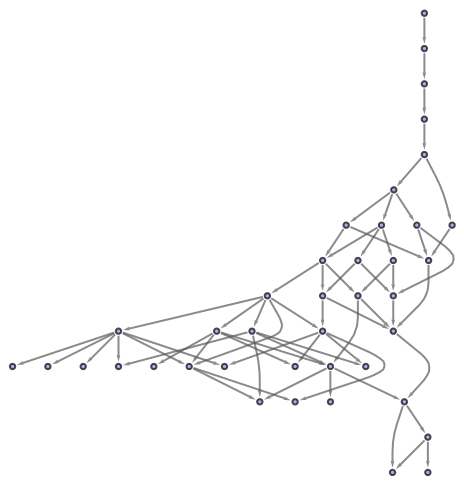In:=Out=In:=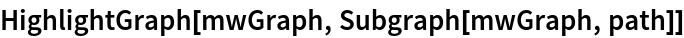Out=